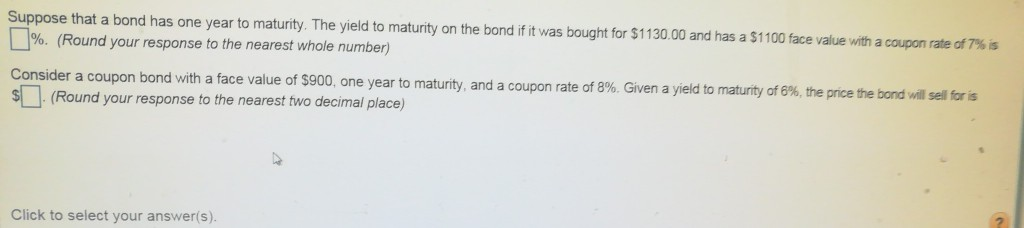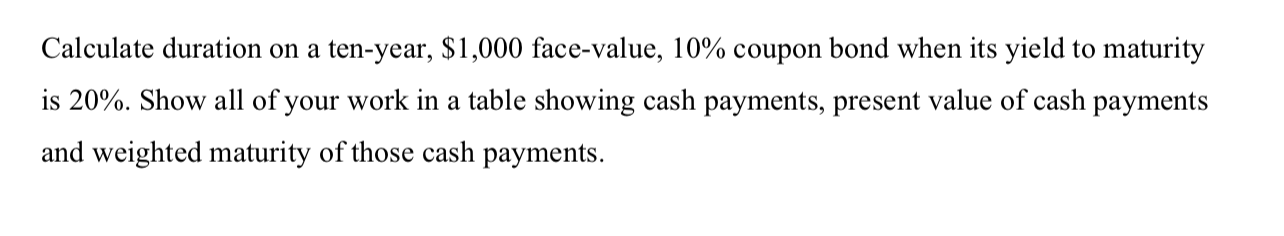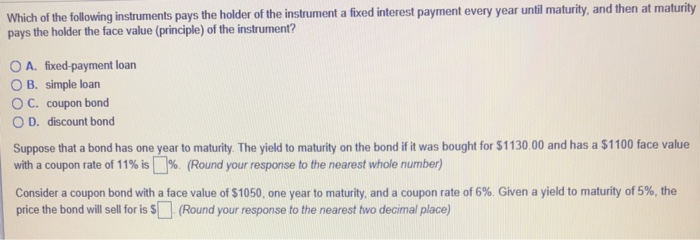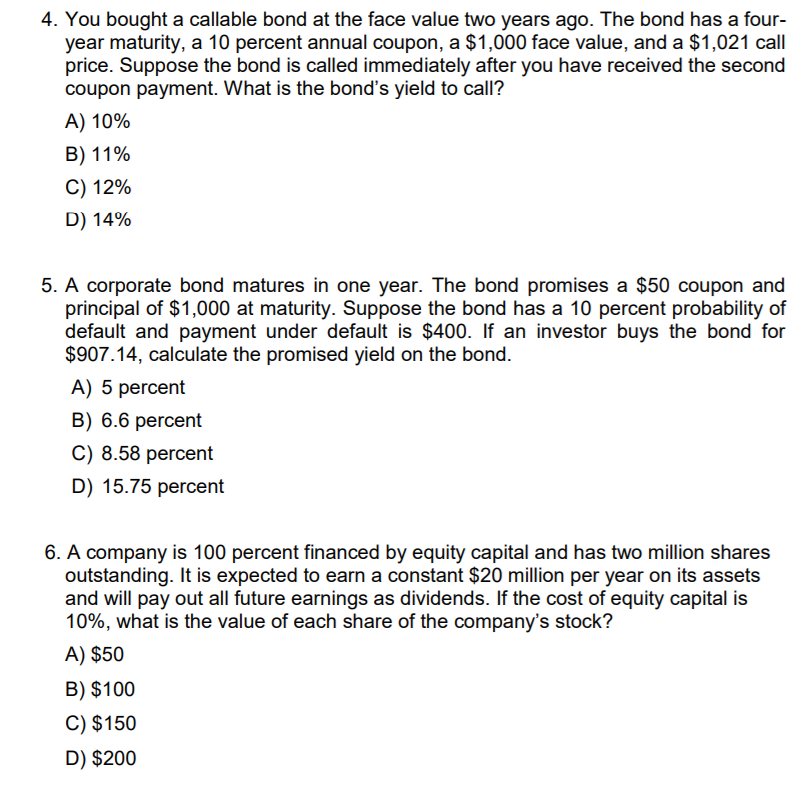Homework Help Question & Answers

# Suppose that a Detroit municipal bond was bought at issue for \$5,000. Its maturity was ten...

Suppose that a Detroit municipal bond was bought at issue for \$5,000. Its maturity was ten years, the face value was \$6,000 and the coupon rate was 5%. a) What was the initial yield to maturity? b) Suppose that in year 5 the coupon was cut to 2% and the face value was cut by \$5,250 due to bankruptcy. What annual return did the bond holder experience? Is it greater than or less than the yield ot maturity? Why? c) If the bond holder could have sold in year four at a price of \$5,100 (after receiving the year 4 coupon) would s/he have been better off than waiting until year 5 and experiencing the bankruptcy (as described in part b))? Explain.

#### Homework Answers

Answer #1

a. The yield to maturity of the bond is calculated as =RATE(nper,pmt,pv.fv) where nper =10 years, pmt = 5%*6000 =\$300, pv= purchase value =5000 and fv -face value =6000

The yield to maturity of the bond =RATE(10,300,-5000,6000) = 7.42%

b. Now, we calculate the price of the bond when there is bankruptcy =PV(rate,nper,pmt,fv) =PV(0.0742,5,120,750) =1010.94

Total return = 300*4+120 + 1010.94 -5000 = -2,669.06 .

prcentage return over 5 year period = -2669.06/5000 =-0.5338

Annualized (1-0.5338)^(1/5) -1 = -0.1415 = -14.15%

(c) If he had sold the bond in year 4, his YTM would be =RATE(6,300,-5100,6000) = 8.27%. So, he would have better better of since he ot a better return

Know the answer?
Your Answer:

#### Post as a guest

Your Name:

What's your source?

#### Earn Coin

Coins can be redeemed for fabulous gifts.

Not the answer you're looking for? Ask your own homework help question. Our experts will answer your question WITHIN MINUTES for Free.
Similar Homework Help Questions
• ### Suppose that a bond has one year to maturity. The yield to maturity on the bond...Suppose that a bond has one year to maturity. The yield to maturity on the bond if it was »%. (Round your response to the nearest whole number) Consider a coupon bond with a face value of \$900, one year to maturity, and a coupon rate of 8% Given a bought for \$1130.00 and has a \$1 100 face value with a coupon rate of 7% yield to maturity of8%the price the bond wil sel tris (Round your response to...

• ### The ten-year bond yields 5.5% and has a coupon of 7.6%. If this yield to maturity...

The ten-year bond yields 5.5% and has a coupon of 7.6%. If this yield to maturity remains unchanged, what will be its price one year hence? Assume annual coupon payments and a face value of \$100

• ### I have a couple Yield to Maturity practice questions I'm working on out of my textbook....

I have a couple Yield to Maturity practice questions I'm working on out of my textbook. Any help appreciated! a.) Suppose that a bond has one year to maturity. What is the yield to maturity on the bond if it was bought for \$1080 and has a \$1300 face value with a coupon rate of 9%? b.) Consider a coupon bond with a face value of \$1500, one year to maturity, and a coupon rate of 6%. With a yield...

• ### Calculate duration on a ten-year, \$1,000 face-value, 10% coupon bond when its yield to maturity is...Calculate duration on a ten-year, \$1,000 face-value, 10% coupon bond when its yield to maturity is 20%. Show all of your work in a table showing cash payments, present value of cash payments and weighted maturity of those cash payments.

• ### A ten-year, zero-coupon bond with a yield to maturity of 6% has a face value of...

A ten-year, zero-coupon bond with a yield to maturity of 6% has a face value of \$1000. An investor purchases the bond when it is initially traded, and then sells it four years later. What is the rate of return of this investment, assuming the yield to maturity does not change? can someone explain step by step

• ### Which of the following instruments pays the holder of the instrument a fixed interest payment every...Which of the following instruments pays the holder of the instrument a fixed interest payment every year until maturity, and then pays the holder the face value (principle) of the instrument? at maturity O A. fixed-payment loan O B. simple loan O C. coupon bond O D. discount bond Suppose that a bond has one year to maturity. The yield to maturity on the bond if it was bought for \$1130.00 and has a \$1100 face value with a coupon...

• ### Bond Prices: On Dec. 30th 2019, the interest rate (yield-to-maturity)on a ten-year Treasury bond was 1.827%....

Bond Prices: On Dec. 30th 2019, the interest rate (yield-to-maturity)on a ten-year Treasury bond was 1.827%. If the bond paid a 2% annual coupon for 10 years and had a par value of \$1,000, what price would it have sold for on Dec. 30th? On Mar. 5nd 2020, the interest rate (yield-to-maturity) on a ten-year Treasury had dropped to 0.926%. Again, if a bond paid a 2% annual coupon for 10 years and had a par value of \$1,000, what...

• ### 4. You bought a callable bond at the face value two years ago. The bond has...4. You bought a callable bond at the face value two years ago. The bond has a four- year maturity, a 10 percent annual coupon, a \$1,000 face value, and a \$1,021 call price. Suppose the bond is called immediately after you have received the second coupon payment. What is the bond's yield to call? A) 10% B) 11% C) 12% D) 14% 5. A corporate bond matures in one year. The bond promises a \$50 coupon and principal of...

• ### The Guilford Corporation has a bond outstanding with a face value of \$1,000 that reaches maturity in ten years. The bond...

The Guilford Corporation has a bond outstanding with a face value of \$1,000 that reaches maturity in ten years. The bond certificate indicates that the stated coupon rate for this bond is 8.0% and that the coupon payments are made semiannually. Assuming the appropriate yield to maturity (YTM) on the Guilford bond is currently 11.1%, then the current price of this bond is closest to a. \$979 b. \$652 c. \$1,142 d. \$816 e. \$438

• ### (a)Suppose a 10 percent coupon, \$1,000 bond with ten years left to maturity is selling for...

(a)Suppose a 10 percent coupon, \$1,000 bond with ten years left to maturity is selling for \$1,200. What is the yield, assuming that interest is paid semi-annually? If, in part (a), the 10 percent coupon was paid quarterly on the bond, what would the bond sell for, given that the effective annual yield remained unchanged? If, in part (a), the 10 percent coupon was paid monthly on the bond, what would the bond sell for, given that the annual yield...

Free Homework App

Scan Your Homework
to Get Instant Free Answers
Need Online Homework Help?

Get Answers For Free
Most questions answered within 3 hours.